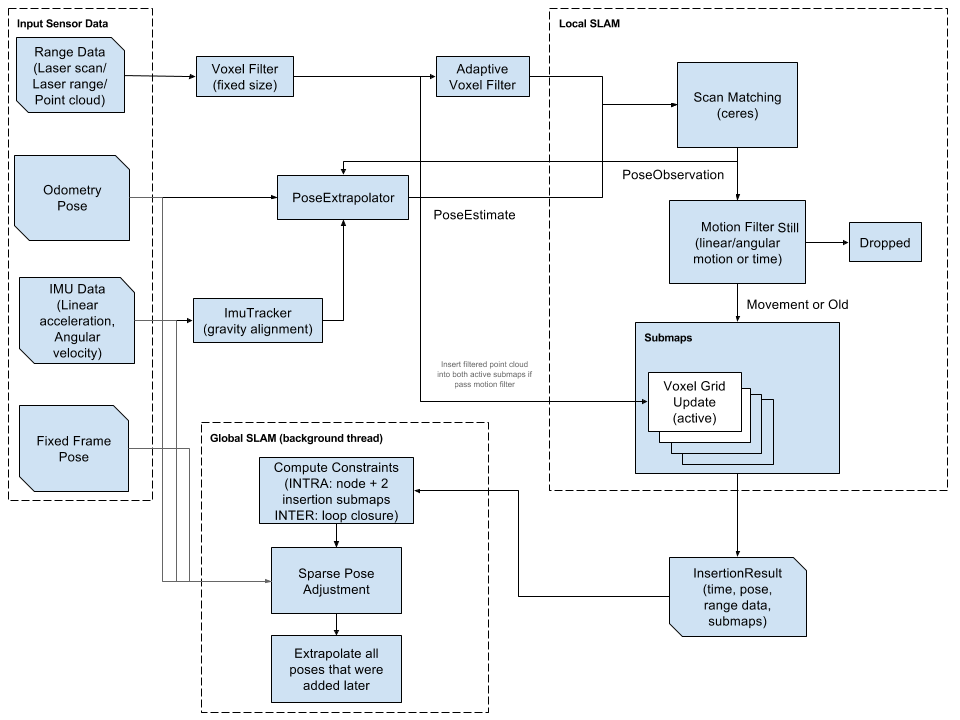## Monday, April 7, 2014

### Particle Swarm Optimization

Since my last post, I have spent a lot of time learning about biologically inspired optimization techniques.  Some commonly used biological machine learning methods include genetic algorithms and neural networks.  However, I decided to dive a little bit deeper and try out a few newer algorithms.  The two algorithms I have been working with are called Particle Swarm Optimization (PSO) and Ant Colony Optimization (ACO).  In this post I will talk about PSO.

Particle Swarm OptimizationThis is a representation of Particle Swarm Optimization.  The solution that is found for a particle is swayed by the global best and the individual particle's best solutions.  http://www.sciencepubco.com/index.php/JACST/article/view/84

PSO is a stochastic technique that is based on the way that swarms move towards a goal.  Each particle is a potential solution (or bee in a swarm) and it moves in some direction with a velocity.  The velocity is determined by a combination of the individual particle's best solution, the global swarm's best solution and an inertia weight.  First developed by Kennedy and Eberhart in 1995, each particle is updated with the equations shown below:http://web.ist.utl.pt/gdgp/VA/pso.htm
The interesting equation above is the velocity update.  Essentially, the new velocity for particle i is the addition of the previous velocity for that particle times the inertia weight (a function of how many iterations we have performed), the randomly weighted difference between the particle and the particle best and the randomly weighted difference between the particle and the global best.  randomly uniform weighting of the differences is a way of introducing unpredictability into the equation so that the system does not get stuck at a local minimum.  The position update just adds the new velocity to the previous position.

The algorithm for performing these actions is fairly straight-forward as well.  The idea is to update each particle and determine if that particle has a better fitness value than it has ever had before, and then determine if it has a better fitness than the swarm's best fitness.  This way, each particle has the equivalent of a unique mind as well as the swarm mind.  Below is the algorithm specifics:

1. A set of N particles are created such that each particle contains random values in the set.
2. It is determined whether each particle is its best case, if it is, particle i's best is this particle.
3. It is determined whether the best particle is the swarms best case, if it is, the swarm best is this particle.
4. Each particle's velocity and position are updated simultaneously with the new particle and swarm bests.
5. If the global best meets the termination condition or the max iterations are reached, stop.  Else, go back to step 2 and repeat.
Using this algorithm, I was able to solve the N-queens problem.  My implementation can be found here, as a new Seeker in the Do-Anything-Machine.  In the N-queens problem, each particle is an array of rows containing a number that corresponds to a column for the position of each queen.  (For an explanation of the N-queens problem, see the previous post).  The fitness function is simply the number of queens that are on the same horizontals, verticals and diagonals of the chess board.  If the fitness of a particle is zero, the solution is correct.

One issue that I have noticed is that it can usually find a local minimum of the system, but does not always find a global minimum.  My solution was to repeat the algorithm multiple times, until the system met the fitness requirement.

Feel free to let me know what you think of my work in the comments.  I am happy to provide links and help to those who are interested.